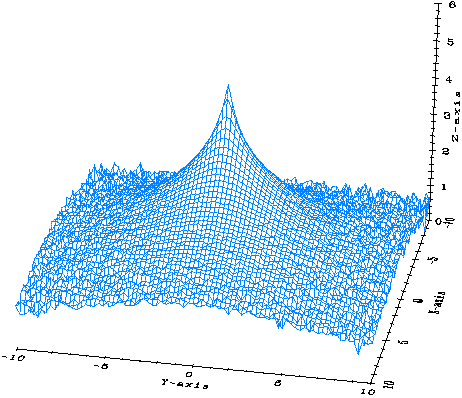## A Study of the Riemann Zeta Function

Khalil Bitar

The well known Riemann zeta function has many interesting properties and has proven to be useful in many applications in physics. In our attempt to use one such property, one described by Voronin's theorems, a new characteristic distribution was discovered numerically and then calculated analytically. This distribution allows the use of the Riemann zeta function as a generator of pseudo random numbers.

The Riemann zeta function is complex and according to Voronin's theorems can approximate any complex number when evaluated for an argument whose real part is in the region 0.5 to 1.0 and for some very large imaginary part. As one increases the imaginary part one will encounter other values where this property will repeat itself. In this `critical strip' if one then chooses a value for the real part of the argument, 0.75 say, and then varies the imaginary part from say 1.0e6 upwards to larger values, these theorems assure us that one will generate for some such value any pre-chosen number with arbitrary precision and that this will happen more than once. We have implemented this process with the aim of studying this characteristic property.

The aim was to determine whether smooth distributions with which complex numbers are generated exist and if so whether they may be calculable. There are several approximations one may use to evaluate the zeta function for an argument with a large imaginary part and we have used what is known as the Seigel formula. The formula involves evaluating a sum of inverse powers with a number of terms that increases with this imaginary part and a remainder involving the gamma function with complex argument. On a typical serial machine this could be programmed to take of the order of a few minutes per evaluation. As one requires a large number of such evaluations to study distributions (of the order of several hundred thousands to few millions) the use of serial machines becomes totally out of the question. On the other hand a massively parallel machine, where many such evaluations may be done concurrently, is ideal. With the CM-2 Connection Machine at SCRI we were able to perform 65536 such evaluations every few minutes, the time being determined by the largest argument used. We could collect and study a few million zeta functions very easily. We have evaluated such sets by taking an initial value for the imaginary part and then incrementing it by a constant `delta.' A typical distribution of the zeta functions generated is given in the figure below. The first interesting property we discovered is that aside from being smooth, these distributions are independent of both the initial value we use and the magnitude of the increment `Delta'. They depend only on the choice of the real part of the argument. Therefore for a particular such value the distribution is universal. Furthermore if one computes the autocorrelations of the numbers generated for any of these distributions this autocorrelation is consistent with zero for delta of order unity or larger. Thus the process may be thought of as a pseudo random number generator.```

```
A three-dimensional plot showing the distribution of a typical set of Riemann zeta functions where the initial imaginary part is incremented by a constant delta.

Using moment integrals over the zeta function we were then able to derive expressions for the distribution of the absolute value of the zeta function and its logarithm. These turn out to be expressible as inverse Mellin transforms over well known functions.

Knowing these distributions allows the use of the zeta function in evaluating the path integrals for quantum mechanical systems. We have tested this on simple systems such as the anharmonic oscillator with good results. Further more since the zeta function is an analytic function with known properties its use in these applications may lead to a definition of the path integral in the continuum.

Dr. Khalil Bitar is a SCRI research scientist in theoretical high energy physics and is a member of the international High Energy Monte Carlo Grand Challenge Collaboration.

archive      tutorial      mystery      new      search      home      contact